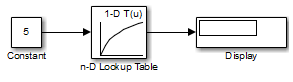Create a Logarithm Lookup Table

Suppose you want to approximate the common logarithm (base 10) over the input range [1, 10] without performing an expensive computation. You can perform this approximation using a lookup table block as described in the following procedure. (If you want to skip the procedure, you can open the model directly.)

1. Copy the following blocks to a Simulink® model:

• One Constant block to input the signal, from the Sources library

• One n-D Lookup Table block to approximate the common logarithm, from the Lookup Tables library

• One Display block to display the output, from the Sinks library

2. Assign the table data and breakpoint data set to the n-D Lookup Table block:

1. In the Number of table dimensions field, enter 1.

2. In the Table data field, enter [0 .301 .477 .602 .699 .778 .845 .903 .954 1].

3. In the Breakpoints 1 field, enter [1:10].

4. Click .

The dialog box looks something like this:3. Double-click the Constant block to open the parameter dialog box, and change the Constant value parameter to 5. Click to apply the changes and close the dialog box.

4. Connect the blocks as follows.5. Start simulation.

The following behavior applies to the n-D Lookup Table block.

Value of the Constant BlockAction by the n-D Lookup Table BlockExample of Block Behavior
Input ValueOutput Value
Equals a breakpointReturns the corresponding output value50.699
Falls between breakpointsLinearly interpolates the output value using neighboring breakpoints7.50.874
Falls outside the range of the breakpoint data setLinearly extrapolates the output value from a pair of values at the end of the breakpoint data set10.51.023

For the n-D Lookup Table block, the default settings for Interpolation method and Extrapolation method are both Linear.